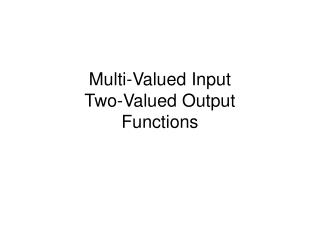DownloadDownload PresentationMulti-Valued Input Two-Valued Output Functions

# Multi-Valued Input Two-Valued Output Functions

Télécharger la présentation## Multi-Valued Input Two-Valued Output Functions

- - - - - - - - - - - - - - - - - - - - - - - - - - - E N D - - - - - - - - - - - - - - - - - - - - - - - - - - -
##### Presentation Transcript

1. Multi-Valued InputTwo-Valued OutputFunctions

2. Example • Automobile features Multi-Valued Input

3. Example • Function Table Multi-Valued Input

4. Definition • A mapping F: P1  P2  Pn is a multi-valued input two-valued output function, Pi = {0,1, … pi-1}, and B = {0,1}. Let X be a variable that takes one value in P = {0,1, … , p-1}. Let S  P. Then XS is a literal of X. When X  S, XS = 1, and when X  S, XS = 0. Let Si Pi, then X1S1 X2S2… XnSn is a logical product. • We can also define minterm and SOP • Any binary function can be represented this way • Problem: Find the SOP for the function given in the previous slide. Multi-Valued Input

5. Bit Representation • Example: Bit representation for the previous example: x1 x2 x3 01-012-012311-100-100011-010-010111-001-001001-110-0001 Multi-Valued Input

6. Restriction Multi-Valued Input

7. Example Multi-Valued Input

8. Restriction • Theorem c F = c F(|c) • The restriction is also called cofactor • What is the relation Shannon’s expansion? Multi-Valued Input

9. Example Multi-Valued Input

10. Tautology • When the logical expression F is equal to 1 for all the input combinations, F is a tautology. The problem of determining whether a given logical expression is a tautology or not is the tautology decision problem. • Example. Which are tautologies? Multi-Valued Input

11. Inclusion Relation • Let F and G be logic functions. For all the minterms c such that F(c) = 1, if G(c) = 1, then F ≤ G, and G contains F. If a logic function F contains a product c, then c is an implicant of F. • Let c be a logical product and F be a logical expression. Then c ≤ F  F(|c) 1. Multi-Valued Input

12. Example • When c2 = (11-010-1101)? Multi-Valued Input

13. Equivalence • The following theorem shows that the determination of the equivalence of two SOPs is transformed to the tautology problem: Multi-Valued Input

14. Divide and Conquer Method (9.1) Multi-Valued Input

15. Divide and Conquer Method • By using the previous theorem, a given SOP is partitioned into k SOPs. In performing some operation on F, first expand F into the form (9.1). Then, for each F(|ci), apply the operation independently. Finally, by combining the results appropriately, we have the results for the operation on F. Since, the same operation can be applied to F(|ci) as to F, this method can be computed by a recursive program. • Definition. Let t(F) be the number of products in an SOP F. Multi-Valued Input

16. Divide and Conquer Methods Multi-Valued Input

17. Selection Method for Variables • Chose variables that have the maximum number of active columns • Among those, chose variables where the total sum of 0’s in the array is maximum • Example Multi-Valued Input

18. Complementation of SOPs Multi-Valued Input

19. Complementation of SOPs • When n = 10, this method is about 100 times faster than using De Morgan’s theorem Multi-Valued Input

20. Example • Find the complement for F Multi-Valued Input

21. Tautology Decision • When there is a variable Xi and at least one constant a  Pi satisfying F(|Xi = a) ≤ F(X1, … , Xi, … , Xn), the function F is weakly unate with respect to the variable Xi. • In an array F, consider the sub-array consisting of cubes that depend on Xi. In the variable Xi in this array, if all the values in a column are 0, then the SOP F is weakly unate with respect to the variable Xi. Multi-Valued Input

22. Example • Consider the F. Is F weakly unate? 1111-1110-11101111-1101-11010110-0110-11010101-0111-1101 Multi-Valued Input

23. Theorems Multi-Valued Input

24. Tautology Decision Multi-Valued Input

25. Generation of Prime Implicants • Let X be a variable that takes a value in P = {0, 1, … , p-1}. If there is a total order () on the values of variable X in function F, such that j  k (j,k  P) implies F(|X=j) ≤ F(|X=k), then the function is strongly unate with respect to X. If F is strongly unate with respect to all variables, then the function F is strongly unate. • Assume that F is an SOP. If there is a total order () among the values of a variable X, and if j  k, then each product term of the SOP F(|X=j) is contained by all the product terms of the SOP F(|X=k). In this case the SOP F is strongly unate with respect to X. • Lemma. If F is strongly unate with respect to Xi, then F is weakly unate with respect to Xi. Multi-Valued Input

26. Generation of Prime Implicants Multi-Valued Input

27. Generation of Prime Implicants Multi-Valued Input

28. The Sharp Operation • Sharp operation (#) and disjoined sharp operation ( # ) compute FG. • Example. Let a = (11-11-11) and b = (01-01-01). Find a#b and a # b • Example. Let B = {b1,b2}, where b1 = (11-11-11) and b2 = (10-10-10). Let C={c1,c2,c3}, where c1 = (10-11-11), c2 = (11-10-11), and c3 = (11-11-10). Find a#C and a # C Multi-Valued Input